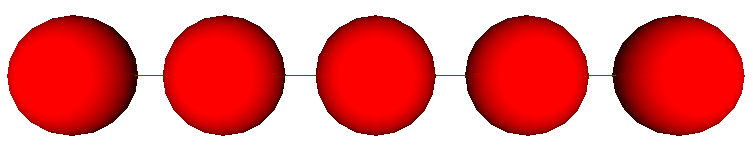basic

# Prerequisites

You must follow the previous tutorial steps before starting this tutorial. You need results of previous tutorial steps. These results will be used as input data for this simulation. More precisely, you need this discrete domainPlease copy one of this domain in the current folder as it follows

Now, your current directory must look like

and the minimal required files are :
and the minimal required files are :

# The input file

The goal of this simulation is to clamp the discrete element at the left and load the discrete element at the right. This simulation can be set through input file only. You can create a new input file named `tensile.inp` with the following input.

# Step by step description of input file

The following plugin loads a discrete domain file into the simulation

The following plugin converts all the `ElementPair` (described in a previous tutorial step) into mechanical beams. To get full description of what this beam are, you can consult the scientific bibliography.

The following plugin computes the optimal time step. It corresponds to the higher time step that ensures stable simulations.

The following plugin clears all the forces and torques applied to elements and bodies. After this plugin the domain is completely relaxed.

The following plugin applies a force on all the discrete elements indexed by the `left` SetOf. Here, only one discrete element is concerned. The `X=...` corresponds to the value of the force along the X axis. A mathematical formula is given that corresponds to : if the iteration is lower than 1,000, the force is 100,000 times the iteration value or zero instead.

The following plugin computes the mechanical induced by the deformation of beams. The given loads are applied to the connected discrete elements.

The following plugin applies the velocity verlet integration scheme that computes linear and angular accelerations, velocities and displacements.

The following plugin imposes displacements to a discrete element set. Here the discrete elements are clamped (they can’t move).

The following plugin checks if the computation is right.

The following plugin saves the current state of the domain all 100 iterations.

# Running example and showing results.

Now, check first if your project is compiled with

Run the example

Show the result

You should see the `granoo3-viewer` window. If you press the Read button, you should see an animation where you domain oscillates.